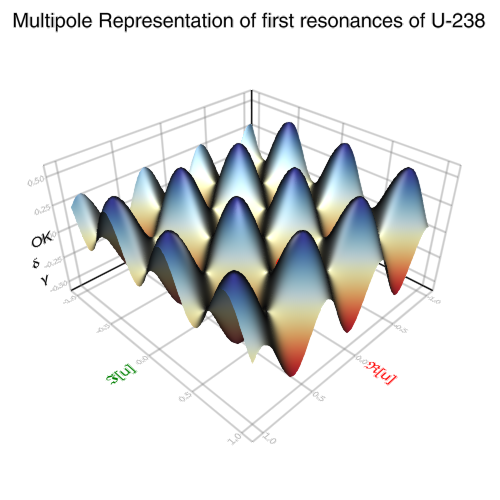## Axis + Surface

```using Makie

vx = -1:0.01:1
vy = -1:0.01:1

f(x, y) = (sin(x*10) + cos(y*10)) / 4
scene = Scene(resolution = (500, 500))
# One way to style the axis is to pass a nested dictionary / named tuple to it.
surface!(scene, vx, vy, f, axis = (frame = (linewidth = 2.0,),))
psurf = scene[end] # the surface we last plotted to scene
# One can also directly get the axis object and manipulate it
axis = scene[Axis] # get axis

# You can access nested attributes likes this:
axis[:names, :axisnames] = ("\\bf{ℜ}[u]", "\\bf{𝕴}[u]", " OK\n\\bf{δ}\n γ")
tstyle = axis[:names] # or just get the nested attributes and work directly with them

tstyle[:textsize] = 10
tstyle[:textcolor] = (:red, :green, :black)
tstyle[:font] = "helvetica"

psurf[:colormap] = :RdYlBu
wh = widths(scene)
t = text!(
campixel(scene),
"Multipole Representation of first resonances of U-238",
position = (wh / 2.0, wh - 20.0),
align = (:center,  :center),
textsize = 20,
font = "helvetica",
raw = :true
)
c = lines!(scene, Circle(Point2f0(0.1, 0.5), 0.1f0), color = :red, offset = Vec3f0(0, 0, 1))
scene
#update surface
# TODO explain and improve the situation here
psurf.converted[] = f.(vx .+ 0.5, (vy .+ 0.5)')
scene

```